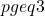# Estimation of parameters of the simple multivariate linear model with student t-errors

This paper considers estimation of the intercept and slope vector parameters of
the simple multivariate linear regression model with Student-t errors in the presence
of uncertain prior information on the value of the unknown slope vector. The
unrestricted, restricted, preliminary test, shrinkage, and positive-rule shrinkage
estimators are defined together with the expressions for the bias, quadratic bias,
quadratic risk and mean squared errors (mse) functions of the estimators are derived.
on the study we conclude that forshrinkage estimators are recommended,
and for, the preliminary test estimators are preferable.